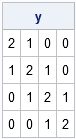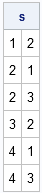Suppose that you want to create a matrix in SAS/IML software that has a special structure, such as a tridiagonal matrix. How do you do it? Or suppose that you want to find elements of a matrix A such that A[i,j] satisfies a certain condition. How do you get the subscripts i and j?

These two questions are related. Each requires specifying where elements are located in a matrix.

### Indices and Subscripts

There are two ways to specify the location of an element in a SAS/IML matrix: by subscripts and by indices.

• Subscripts are used when you are manipulating all or part of a row or column, or manipulating a rectangular submatrix.
• Indices are used in the more general case where you want to retrieve or assign values that are scattered throughout the matrix.

Mathematicians and statisticians often specify subscripts. For example, A[2,1] is the element of A that is in the second row and first column.

Statistical programmers need to also work with indices. Because SAS/IML software stores matrices in row-major order, the first element of A is A, the second is A, the third is A, and so forth. However, notice that you do not know the subscripts for A unless you know the shape of A. If A is a 3x3 matrix, A corresponds to A[1,3]. However, if A is a 2x2 matrix , A corresponds to A[2,1].

Consequently, in order to convert indices of a matrix to subscripts (and the other way around), you need to know the number of columns in the matrix.

Update: As of SAS/IML 12.1, the SAS/IML language includes the NDX2SUB function and the SUB2NDX function. There is no longer a need to implement this functionality yourself.

### Converting Subscripts to Indices

Suppose that the number of columns in a matrix is p. The index of the element that is located in row i and column j is j + p*(i-1). You can use this fact to write a SAS/IML module that returns the indices that correspond to a set of subscripts:

```proc iml; /** For an nxp matrix, this module returns the indices (1<=idx<=n*p) that correspond to the subscripts. The subscripts are passed in via a kx2 matrix. Each row is an (i,j) coordinate. **/ start sub2ind( p, sub ); idx = sub[,2] + p * (sub[,1]-1); return ( idx ); finish;```

You can test the module by using it to construct a tridiagonal matrix that contains 2s on the diagonal and 1s on the sub- and superdiagonals. The following SAS/IML program uses the DIAG function to construct a diagonal matrix. It then computes the subscripts of the superdiagonal (which, for this example, are (1,2), (2,3), and (3,4)) and the subdiagonal (which are (2,1), (3,2), and (4,3)). The sub2ind module converts these subscripts to indices, and the value 1 is assigned to all of the corresponding elements of the matrix.

```/** construct a tridiagonal matrix **/ y = diag({2,2,2,2}); p = ncol(y); superDiag = T(1:p-1) || T(2:p); subDiag = T(2:p) || T(1:p-1); /** assign all super- and subdiagonal elements at once **/ idx = sub2ind(p, superDiag//subDiag); y[idx] = 1; /** no loop! **/ print y;```If you use tridiagonal matrices often, you can encapsulate these statements into a SAS/IML module.

### Converting Indices to Subscripts

It is not common to need to convert indices to subscripts. The main advantage of subscripts is to the programmer: they can help you to debug code or to understand the structure of a large matrix that cannot be easily printed. For example, if you have a huge sparse matrix, you can use the LOC function to find the indices of all nonzero elements, and then convert those indices to subscripts so that you can examine the structure of the nonzero elements.

The following SAS/IML module converts indices to subscripts. It uses modular arithmetic to convert an index to a column and row number.

```/** For an nxp matrix, this module returns the subscripts that correspond to the indices (1<=idx<=n*p). The indices are passed in via a kx1 or 1xk vector, ind. The module returns a kx2 matrix, where each row is an (i,j) coordinate. **/ start ind2sub( p, ind ); idx = colvec(ind); n = nrow(idx); col = 1 + mod(idx-1, p); row = 1 + (idx-col) / p; return ( row || col ); finish;```

You can use the module to display the rows and columns of elements that satisfy a certain condition. For example, the following statements locate all of the even numbers in a matrix:

```x = {1 2 3, 4 5 6, 7 8 9, 10 11 12}; idx = loc( mod(x, 2)=0 );```

What are the subscripts of the missing elements? Call the ind2sub module to find out:

```s = ind2sub(ncol(x), idx); print s;```Share